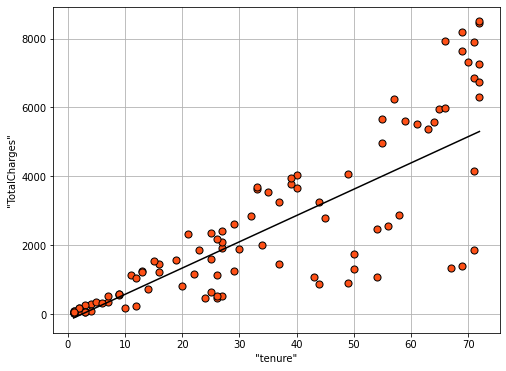### VerticaPy

Python API for Vertica Data Science at Scale

# Regression¶

Regressions are machine learning algorithms used to predict numerical response columns. Predicting the salaries of employees using their age or predicting the number of cyber attacks a website might face would be examples of regressions. The most popular regression algorithm is the linear regression.

You must always verify that all the assumptions of a given algorithm are met before using them. For example, to create a good linear regression model, we need to verify the Gauss-Markov assumptions.

• Linearity : the parameters we are estimating using the OLS method must be linear.
• Non-Collinearity : the regressors being calculated aren’t perfectly correlated with each other.
• Exogeneity : the regressors aren’t correlated with the error term.
• Homoscedasticity : no matter what the values of our regressors might be, the error of the variance is constant.

Most of regression models are sensitive to unnormalized data, so it's important to normalize and decompose your data before using them (though some models like random forest can handle unnormalized and correlated data). If we don't follow the assumptions, we might get unexpected results (example: negative R2).

Let's predict the total charges of the Telco customers using their tenure. We will start by importing the telco dataset.

In :
```import verticapy as vp
```

Next, we can import a linear regression model.

In :
```from verticapy.learn.linear_model import LinearRegression
```

Let's create a model object. Since Vertica has its own model management system, we just need to choose a model name. The model will be created in a schema. The default schema is 'public'.

In :
```model = LinearRegression("LR_churn")
```

We can then fit the model with our data.

In :
```model.fit(churn, ["tenure"], "TotalCharges")
model.plot()
```
Out:
`<AxesSubplot:xlabel='"tenure"', ylabel='"TotalCharges"'>`We have many metrics to evaluate the model.

In :
```model.report()
```
Out:value explained_variance 0.682078535751238 max_error 3997.15535368371 median_absolute_error 480.052176277521 mean_absolute_error 879.836040491484 mean_squared_error 1633328.42507227 root_mean_squared_error 1278.0173805830145 r2 0.682078535751241 r2_adj 0.6820333122143635 aic 100604.71116768524 bic 100618.42591335099
Rows: 1-10 | Columns: 2

Our example forgoes splitting the data into training and testing, which is important for real-world work. Our main goal in this lesson is to look at the metrics used to evaluate regressions. The most famous metric is R2: generally speaking, the closer R2 is to 1, the better the model is.

In the next lesson, we'll go over classification models.